# Roll the dice

What is the probability that if we roll the dice, a number less than 5 falls?

p =  0.6667

### Step-by-step explanation:

$p=4\mathrm{/}6=0.6667$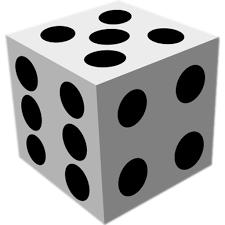Did you find an error or inaccuracy? Feel free to write us. Thank you!Tips to related online calculators
Would you like to compute count of combinations?

## Related math problems and questions:

• One dice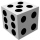Calculate the probability of one dice roll with the numbers 1, 2, 3, 4, 5, 6 on the walls. Write the results in a notebook in the shape of a fraction in the basic form: 2/3. a, The number 1 falls on the cube. b, The number 5 falls on the cube. c, An even
• The diceWhat is the probability of events that if we throw a dice is rolled less than 6?
• Three dice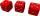What is the probability that when we roll three dice, we roll the numbers 1,2,3?
• Two diceWe roll two dice. What is the probability that the sum of the falling numbers is greater than 3?
• We rollWe roll two dice A. - what is the probability that the sum of the falling numbers is at most 4 B. - is at least 10 C. - is divisible by 5?
• Unknown number 5I think of an unknown number. If we enlarge it five times then subtract 3 and the result decreases by 75% we get one greater than the number. What number am I thinking of?
• One threeWe throw two dice. What is the probability that max one three falls?
• The diceFind the probability that a number divisible by two or three will fall when the dice are rolled.
• Sum on diceWe have two dice. What is the greater likelihood of fall a total sum 7 or 8 ? (write 7, 8 or 0 if the probabilities are the same)?
• NumbersDetermine the number of all positive integers less than 4183444 if each is divisible by 29, 7, 17. What is its sum?
• Dice 7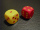A standard number cube is tossed 210 times. What is a reasonable prediction for the number of times the number cube will land on a 5?
• Perpetrator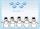The perpetrator is a number that is smaller than the number 80. It is a multiple of five and it is odd. If we added up the tens and ones of this number we get number 8.
• DiceWe throw five times the dice. What is the probability that six fits exactly twice?
• DicesWe will throw two dice. What is the probability that the ratio between numbers on first and second dice will be 1:2?
• Dice and coinA number cube is rolled and a coin is tossed. The number cube and the coin are fair. What is the probability that the number rolled is greater than 2 and the coin toss is head?
• Six on diceWhat is the probability that when throwing two dice will fall at least one six?
• Scrap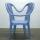From 6 products are 3 scrap. What is the probability that the random pick of 2 products have no defective product?